Overdetermined and underdetermined systems of equations put simply

This article aims at providing real world examples and links for overdetermined and undetermined systems of equations. Before starting, we will suppose that all over and underdetermined systems are obtained from square systems which admit one and only one solution (i.e. comes from a coefficient matrix with non zero determinant).

Overdetermined systems

When a system of linear equations has more equations than unknowns, we say it is overdetermined. It means what it says: too many rules at once are being imposed, and some of them may be conflicting. Still, it is false to say that an overdetermined system does not have any solutions: it may or it may not.

Intuition

Intuitively, we can think of a system of equations as a set of requests. Imagine you have a group ofpeople in front of you (the unknowns), and you are supposed to give each person something specific to do. If you give more commands than the number of people, then we have an overdetermined system. It is clear that when this happens, at least one person must have received more than one command. However, there are two possible scenarios.

Quick method to find line of shortest distance for skew lines

In linear algebra it is sometimes needed to find the equation of the line of shortest distance for two skew lines. What follows is a very quick method of finding that line.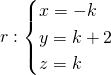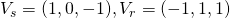So they clearly aren’t parallel. They aren’t incidental as well, because the only possible intersection point is for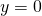, but when,is at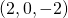, which doesn’t belong to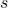. It does indeed make sense to look for the line of shortest distance between the two, confident that we will find a non-zero result.